1. The energy-level diagram for an atom that has four energy states is shown.What is the number of different wavelengths in the emission spectrum of this atom?

2. A graph of the variation of average binding energy per nucleon with nucleon number has a maximum. What is indicated by the region around the maximum?
3. A detector, placed close to a radioactive source, detects an activity of 260 Bq. The average background activity at this location is 20 Bq. The radioactive nuclide has a half-life of 9 hours.

What activity is detected after 36 hours?

4. Kindly refer the question below:

The average binding energy per nucleon of the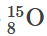nucleus is 7.5 MeV. What is the total energy required to separate the nucleons of one nucleus of?

5. The half-life of a radioactive element is 5.0 days. A freshly-prepared sample contains 128 g of this element. After how many days will there be 16 g of this element left behind in the sample?
6. Atomic spectra are caused when a certain particle makes transitions between energy levels.

What is this particle?

7. A sample contains an amount of radioactive material with a half-life of 3.5 days. After 2 weeks the fraction of the radioactive material remaining is
8. In a nuclear fission reaction, nucleus X splits into nucleus Y and nucleus Z. Which of the following gives a possible order of the nuclei from lowest to highest binding energy per nucleon?
9. Kindly refer the question below:

Bismuth-210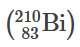is a radioactive isotope that decays as follows.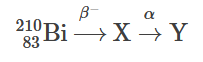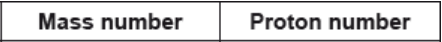10. The initial number of atoms in a pure radioactive sample is N. The radioactive half-life of the sample is defined as the
11. Kindly refer the question below:

Which of the following is true about beta minus () decay?

12. Geiger and Marsden bombarded a thin gold foil with alpha particles. They observed that a small fraction of the alpha particles were deflected through angles greater than 90˚. What does this observation suggest about the nucleus?
13. Kindly refer the question below:

The nuclear reaction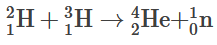would best be described as

14. What is the definition of the unified atomic mass unit?
15. Which of the following affects the rate at which a sample of a radioactive material decays?
16. Nucleus P decays by a sequence of emissions to form nucleus Q.

One  particle and two β particles are emitted during the sequence. Which statement is correct?

17. Kindly refer the question below:

Which of the following correctly identifies the three particles emitted in the decay of the nucleus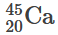into a nucleus of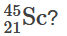18. Kindly refer the question below:

The rest mass of a proton is . The energy of a proton at rest is

19. A simple model of the hydrogen atom suggests that the electron orbits the proton. What is the force that keeps the electron in orbit?
20. The Geiger–Marsden experiment provides evidence for
21. Emission and absorption spectra provide evidence for
22. Data concerning nuclides are plotted using the axes below.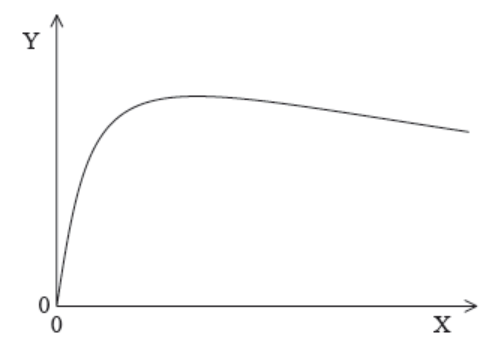What are the axis labels for this graph?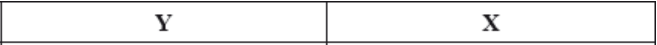23. What is the relationship between nucleon number A, proton number Z and neutron number N?
24. The nuclear reaction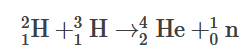is an example of

25. A radioactive isotope has a half-life of two minutes. A sample contains sixteen grams of the isotope. How much time elapses until one gram of the isotope remains?
26. Kindly refer the question below:

When an alpha particle collides with a nucleus of nitrogen-14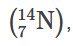a nucleus X can be produced together with a proton. What is X?

27. Kindly refer the question below:

The binding energy per nucleon of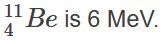What is the energy required to separate the nucleons of this nucleus?

28. Which of the following is true in respect of both the Coulomb interaction and the strong interaction between nucleons in an atom?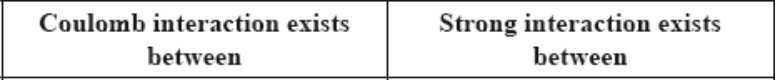29. Kindly refer the question below:

A radioactive sample has activity A0 at t=0. What will be the activity of the sample after two half-lives?

30. Kindly refer the question below:

Element X decays through a series of alpha (α) and beta minus (β) emissions. Which series of emissions results in an isotope of X?

31. Kindly refer the question below:

Which Feynman diagram shows beta-plus (β+) decay?

32. A radio-isotope has an activity of 400 Bq and a half-life of 8 days. After 32 days the activity of the sample is
33. As quarks separate from each other within a hadron, the interaction between them becomes larger. What is the nature of this interaction?
34. Three of the fundamental forces between particles are

I.     strong nuclear

II.     weak nuclear

III.     electromagnetic.

What forces are experienced by an electron?

35. Which of the following lists three fundamental forces in increasing order of strength?
36. The relationship between proton number Z, neutron number N and nucleon number A is
37. Kindly refer the question below:

The mass defect for deuterium is 4×10–30 kg. What is the binding energy of deuterium?

38. What is the energy equivalent to the mass of one proton?
39. A simple model of an atom has five energy levels. What is the maximum number of different frequencies in the emission spectrum of that atom?
40. In the Geiger–Marsden experiment α-particles are scattered by gold nuclei. The experimental results provide evidence that
41. Kindly refer the question below:

The binding energy per nucleon of a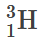nucleus is 3 MeV. What is the minimum energy needed to
completely separate the nucleons of.

42. Two pure samples of radioactive nuclides X and Y have the same initial number of atoms.

The half-life of X is After a time equal to 4 half-lives of X the ratio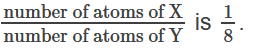What is the half-life of Y?

43. Kindly refer the question below:

The number of neutrons and the number of protons in a nucleus of an atom of the isotope of uranium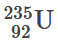are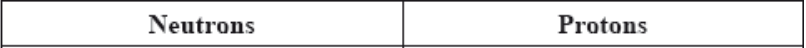44. What is the definition of the unified atomic mass unit?
45. Which particle is acted on by both the strong nuclear force and the Coulomb force?